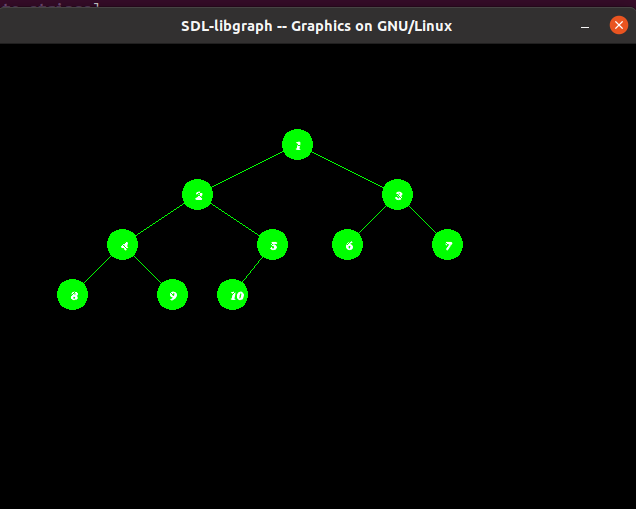# Represent Tree using graphics in C/C++

Prerequisite: graphics.h, How to include graphics.h?

In C/C++ there is graphics.h header file which is used to create the object like line, circle, etc.
Given an array arr[] of N integers, the task is to write C++ program to create the Tree using graphics.h.

Approach: To run the program we have the include the below header file:

```#include
```

We will create a Tree with the help below functions:

1. setcolor(color): This function present in graphic.h header file which is used to set the current drawing color to the new color.
2. floodfill(pattern, color): function is used to fill an enclosed area. The current fill pattern and fill color is used to fill the area.
3. circle(x, y, radius): The header file graphics.h contains circle() function which draws a circle with center at (x, y) and given radius.
4. outtextxy(): The header file graphics.h contains outtextxy() function which displays the text or string at a specified point (x, y) on the screen.

Below is the implementation of to draw Tree using graphics in C++:

## C++

 `// C++ program to draw the tree` `// in graphics.h` `#include ` `#include ` `#include ` `#include ` `using` `namespace` `std;`   `// Function that prints Tree using` `// functions graphic.h header file` `void` `printTree(``int` `x, ``int` `y, ``int``* array,` `               ``int` `index,` `               ``int` `total_elements)` `{`   `    ``// Base Case` `    ``if` `(index >= total_elements)` `        ``return` `NULL;`   `    ``// Convert int value into string` `    ``ostringstream str1;` `    ``str1 << array[index];`   `    ``string str2 = str1.str();` `    ``char``* str = &str2[0u];`   `    ``// Set color of the boundary of` `    ``// circle as green` `    ``setcolor(GREEN);`   `    ``// Draw the circle of radius 15` `    ``// that represent node of Tree` `    ``circle(x, y, 15);` `    ``floodfill(x, y, GREEN);`   `    ``// Print the values of the node` `    ``// in the circle` `    ``outtextxy(x - 2, y - 3, str);`   `    ``// Set the color of the line` `    ``// from parent to child as green` `    ``setcolor(GREEN);`   `    ``// Evaluating left and right child` `    ``int` `left = 2 * index + 1;` `    ``int` `right = 2 * index + 2;`   `    ``// Recursively draw the left subtree` `    ``// and the right subtree` `    ``printTree(x - y / (index + 1), y + 50,` `              ``array, left, total_elements);`   `    ``printTree(x + y / (index + 1), y + 50,` `              ``array, right, total_elements);`   `    ``// Draw the line (or link) when the` `    ``// node is not the leaf node` `    ``if` `(left < total_elements) {` `        ``line(x, y, x - y / (index + 1), y + 50);` `    ``}`   `    ``if` `(right < total_elements) {` `        ``line(x, y, x + y / (index + 1), y + 50);` `    ``}`   `    ``return` `NULL;` `}`   `// Driver Code` `int` `main()` `{` `    ``// Initilize graphic driver` `    ``int` `gd = DETECT, gm;` `    ``initgraph(&gd, &gm, ``"None"``);`   `    ``// Consider the tree as represented` `    ``/*` `             ``1` `          ``/     \` `         ``2       3` `       ``/  \     / \` `      ``4   5    6   7` `     ``/ \  /` `     ``8  9 10` `    ``*/`   `    ``// Given array arr[]` `    ``int` `array[] = { 1, 2, 3, 4, 5,` `                    ``6, 7, 8, 9, 10 };`   `    ``// Function Call` `    ``printTree(300, 100, array, 0, 10);` `    ``getch();`   `    ``// closegraph function closes the` `    ``// graphics mode and deallocates` `    ``// all memory allocated by` `    ``// graphics system` `    ``closegraph();` `}`

Output:Attention reader! Don’t stop learning now. Get hold of all the important DSA concepts with the DSA Self Paced Course at a student-friendly price and become industry ready.

My Personal Notes arrow_drop_upCheck out this Author's contributed articles.

If you like GeeksforGeeks and would like to contribute, you can also write an article using contribute.geeksforgeeks.org or mail your article to contribute@geeksforgeeks.org. See your article appearing on the GeeksforGeeks main page and help other Geeks.

Please Improve this article if you find anything incorrect by clicking on the "Improve Article" button below.

Improved By : arpit7714

Article Tags :
Practice Tags :

1

Please write to us at contribute@geeksforgeeks.org to report any issue with the above content.